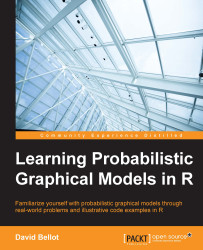•#### Learning Probabilistic Graphical Models in R#### Overview of this book

Probabilistic graphical models (PGM, also known as graphical models) are a marriage between probability theory and graph theory. Generally, PGMs use a graph-based representation. Two branches of graphical representations of distributions are commonly used, namely Bayesian networks and Markov networks. R has many packages to implement graphical models. We’ll start by showing you how to transform a classical statistical model into a modern PGM and then look at how to do exact inference in graphical models. Proceeding, we’ll introduce you to many modern R packages that will help you to perform inference on the models. We will then run a Bayesian linear regression and you’ll see the advantage of going probabilistic when you want to do prediction. Next, you’ll master using R packages and implementing its techniques. Finally, you’ll be presented with machine learning applications that have a direct impact in many fields. Here, we’ll cover clustering and the discovery of hidden information in big data, as well as two important methods, PCA and ICA, to reduce the size of big problems.
Learning Probabilistic Graphical Models in RCreditswww.PacktPub.comPrefaceFree Chapter
Probabilistic ReasoningExact InferenceLearning ParametersBayesian Modeling – Basic ModelsApproximate InferenceBayesian Modeling – Linear ModelsProbabilistic Mixture ModelsAppendixIndex## Building graphical models

The design of a graphical model usually takes into consideration two different aspects. First of all, we need to identify the variables involved in the model. The variables can refer to facts we can observe or measure, such as a temperature, a price, a distance, a number of items, an interval of time, or any other value. A variable can also represent a simple fact that can be true or false.

At the same time, and this is why we're building such models, the variables can capture parts of the problem that we cannot directly measure or estimate but that are related to the problem. For example, a physician is able to see and measure a set of symptoms for a patient. However diseases are not facts that can be directly observed. They can only be deduced from the observation of several symptoms. Let's take the common cold. It is natural to say that someone has a cold, and in practice everybody understands what it means. However, there is no such thing such as a cold, but...